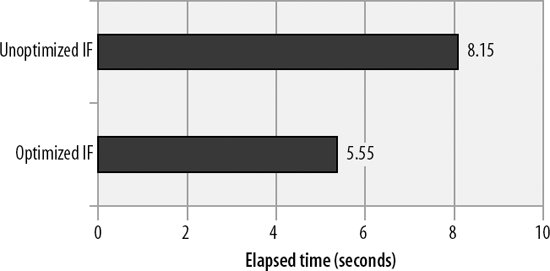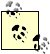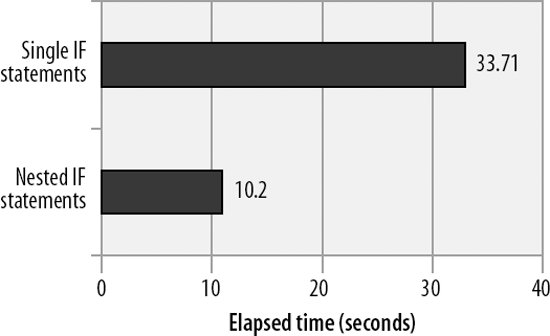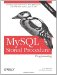# IF and CASE Statements

Another category of statement that is highly amenable to code optimization is the conditional statement categoryIF and CASE statements. This is especially true if these statements are called repetitively within a loop. The essence of optimizing conditional statements like IF and CASE is to reduce the number of comparisons that are performed. You can do this by:

• Testing for the more likely matches earlier in the IF or CASE statement
• Stopping the comparison process as early as possible

22.6.1. Test for the Most Likely Conditions First

When constructing IF and CASE statements, try to minimize the number of comparisons that these statements are likely to make by testing for the most likely scenarios first. For instance, consider the IF statement shown in Example 22-14. This statement maintains counts of various percentages. Assuming that the input data is evenly distributed, the first IF condition (percentage>95) will match about once in every 20 executions. On the other hand, the final condition will match in three out of four executions. So this means that for 75% of the cases, all four comparisons will need to be evaluated.

Example 22-14. Poorly constructed IF statement

 ``` IF (percentage>95) THEN SET Above95=Above95+1; ELSEIF (percentage >=90) THEN SET Range90to95=Range90to95+1; ELSEIF (percentage >=75) THEN SET Range75to89=Range75to89+1; ELSE SET LessThan75=LessThan75+1; END IF; ```

Example 22-15 shows a more efficiently formed IF statement. In this variation, the first condition will evaluate as true in the majority of executions and no further comparisons will be necessary.

Example 22-15. Optimized IF statement

 ``` IF (percentage<75) THEN SET LessThan75=LessThan75+1; ELSEIF (percentage >=75 AND percentage<90) THEN SET Range75to89=Range75to89+1; ELSEIF (percentage >=90 and percentage <=95) THEN SET Range90to95=Range90to95+1; ELSE SET Above95=Above95+1; END IF; ```

Figure 22-7 shows the performance improvement gained by reordering the IF statement so that the most commonly satisfied condition is evaluated first.

Figure 22-7. Effect of optimizing an IF statement by reordering comparisonsIf an IF statement is to be executed repeatedly, placing the most commonly satisfied condition earlier in the IF structure may optimize performance.

22.6.2. Avoid Unnecessary Comparisons

Sometimes an IF or CASE statement will be constructed that has some kind of common condition in each comparison clause. For instance, in Example 22-16, each of the expressions in the IF statement includes an employee_status='U' condition. Even if the employee_status is not equal to "U", each of these comparisons will need to be evaluatedadding some processing overhead.

Example 22-16. IF statement with common condition in each expression

 ```IF (employee_status='U' AND employee_salary>150000) THEN SET categoryA=categoryA+1; ELSEIF (employee_status='U' AND employee_salary>100000) THEN SET categoryB=categoryB+1; ELSEIF (employee_status='U' AND employee_salary<50000) THEN SET categoryC=categoryC+1; ELSEIF (employee_status='U') THEN SET categoryD=categoryD+1; END IF; ```

Example 22-17 shows a more optimized IF structure. In this example, the employee_status is checked first and thenonly if employee_status='U'are the additional comparisons are evaluated. Figure 22-8 demonstrates the optimization.

Example 22-17. Nested IF statement to avoid redundant comparisons

 ```IF (employee_status='U') THEN IF (employee_salary>150000) THEN SET categoryA=categoryA+1; ELSEIF (employee_salary>100000) THEN SET categoryB=categoryB+1; ELSEIF (employee_salary<50000) THEN SET categoryC=categoryC+1; ELSE SET categoryD=categoryD+1; END IF; END IF; ```

To be honest, under most circumstances, tuning IF statements will not greatly improve the performance of your code. The overhead of SQL processing will usually dominate overall execution time. Consequently, we suggest that when it comes to conditional statements, you should prioritize writing readable and maintainable code. If a particular IF statement becomes a bottleneck, then you should consider a rewrite that will improve performance even at the expense of maintainability.

Figure 22-8. Effect of nesting an IF statement to eliminate redundant comparisonsIf your IF or CASE statement contains compound expressions with redundant comparisons, consider nesting multiple IF or CASE statements to avoid redundant processing.

22.6.3. CASE Versus IF

We wondered if there was any performance difference between a CASE statement and an equivalent IF statement. We thought that CASE might be more optimal for comparing a variable against a range of set values, so we speculated that this statement:

``` CASE customer_code
WHEN 1 THEN
SET process_flag=7;
WHEN 2 THEN
SET process_flag=9;
WHEN 3 THEN
SET process_flag=2;
ELSE
SET process_flag=0;
END CASE;
```

might be more efficient than the equivalent IF statement:

``` IF customer_code= 1 THEN
SET process_flag=7;
ELSEIF customer_code= 2 THEN
SET process_flag=9;
ELSEIF customer_code=3 THEN
SET process_flag=2;
ELSE
SET process_flag=0;
END IF;
```

In fact, the opposite turned out to be true. The IF statement is roughly 15% faster than the equivalent CASE statementpresumably this is the result of a more efficient internal algorithm for IF in the MySQL code.

As noted earlier, we advise you to structure your stored program's statements primarily for readability and maintainability, since it is almost always the elapsed time of SQL statements that dominates performance. However, if performance is critical, you may want to make a habit of using IF statements rather than CASE statements in your code.MySQL Stored Procedure Programming
ISBN: 0596100892
EAN: 2147483647
Year: 2004
Pages: 208